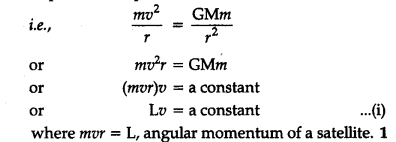# Viscous force increase the velocity of satellite. Explain

When a satellite of mass m revolves in a circular orbit of radius r, around the earth of mass m, the gravitational attraction of earth provides the required centripetal force .According to law of conservation of momentum if no external torque acts on the satellite, then its angular momentum L is conserved. Since, the air friction will provide a retarding torque to satellite, therefore, there will be decrease in angular momentum of the satellite in air.^As a result of it, the velocity of satellite increase according to relation